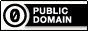# 中文姓名產生器

``````鄭珍慧

``````

Javascript 版：

``````
function random_chinese_name() {
function random(a, l) {
var x = [];
x.push(a[Math.ceil(Math.random() * a.length)]);
while(l > 1) {
x.push(a[Math.ceil(Math.random() * a.length)]);
l--;
}
return x.join("");
}

return random("李 王 張 劉 陳 楊 黃 趙 周 吳 徐 孫 朱 馬 胡 郭 林 何 高 梁 鄭 羅 宋 謝 唐 韓 曹 許 鄧 蕭 馮 曾 程 蔡 彭 潘 袁 於 董 餘 蘇 葉 呂 魏 蔣 田 杜 丁 沈 姜 範 江 傅 鐘 盧 汪 戴 崔 任 陸 廖 姚 方 金 邱 夏 譚 韋 賈 鄒 石 熊 孟 秦 閻 薛 侯 雷 白 龍 段 郝 孔 邵 史 毛 常 萬 顧 賴 武 康 賀 嚴 尹 錢 施 牛 洪 龔".split(" ")) + random("世 中 仁 伶 佩 佳 俊 信 倫 偉 傑 儀 元 冠 凱 君 哲 嘉 國 士 如 娟 婷 子 孟 宇 安 宏 宗 宜 家 建 弘 強 彥 彬 德 心 志 忠 怡 惠 慧 慶 憲 成 政 敏 文 昌 明 智 曉 柏 榮 欣 正 民 永 淑 玉 玲 珊 珍 珮 琪 瑋 瑜 瑞 瑩 盈 真 祥 秀 秋 穎 立 維 美 翔 翰 聖 育 良 芬 芳 英 菁 華 萍 蓉 裕 豪 貞 賢 郁 鈴 銘 雅 雯 霖 青 靜 韻 鴻 麗 龍".split(" "), Math.ceil(Math.random()*2));
}
``````
Ruby 版：
``````
def random_chinese_name
%w[李 王 張 劉 陳 楊 黃 趙 周 吳 徐 孫 朱 馬 胡 郭 林 何 高 梁 鄭 羅 宋 謝 唐 韓 曹 許 鄧 蕭 馮 曾 程 蔡 彭 潘 袁 於 董 餘 蘇 葉 呂 魏 蔣 田 杜 丁 沈 姜 範 江 傅 鐘 盧 汪 戴 崔 任 陸 廖 姚 方 金 邱 夏 譚 韋 賈 鄒 石 熊 孟 秦 閻 薛 侯 雷 白 龍 段 郝 孔 邵 史 毛 常 萬 顧 賴 武 康 賀 嚴 尹 錢 施 牛 洪 龔].shuffle.first +
%w[世 中 仁 伶 佩 佳 俊 信 倫 偉 傑 儀 元 冠 凱 君 哲 嘉 國 士 如 娟 婷 子 孟 宇 安 宏 宗 宜 家 建 弘 強 彥 彬 德 心 志 忠 怡 惠 慧 慶 憲 成 政 敏 文 昌 明 智 曉 柏 榮 欣 正 民 永 淑 玉 玲 珊 珍 珮 琪 瑋 瑜 瑞 瑩 盈 真 祥 秀 秋 穎 立 維 美 翔 翰 聖 育 良 芬 芳 英 菁 華 萍 蓉 裕 豪 貞 賢 郁 鈴 銘 雅 雯 霖 青 靜 韻 鴻 麗 龍].shuffle.first(1 + rand(2)).join("")
end
``````
Perl 版：
``````
sub random_chinese_name {
my \$random = sub {
\$_ = shift;
if (\$_ > 0) { \$_ = 1+rand(\$_) }
else { unshift(@_, \$_); \$_ = 1 }
join("", map { \$_[rand(1+\$#_)] } 1..\$_);
};

\$random->(qw(李 王 張 劉 陳 楊 黃 趙 周 吳 徐 孫 朱 馬 胡 郭 林 何 高 梁 鄭 羅 宋 謝 唐 韓 曹 許 鄧 蕭 馮 曾 程 蔡 彭 潘 袁 於 董 餘 蘇 葉 呂 魏 蔣 田 杜 丁 沈 姜 範 江 傅 鐘 盧 汪 戴 崔 任 陸 廖 姚 方 金 邱 夏 譚 韋 賈 鄒 石 熊 孟 秦 閻 薛 侯 雷 白 龍 段 郝 孔 邵 史 毛 常 萬 顧 賴 武 康 賀 嚴 尹 錢 施 牛 洪 龔)) . \$random->(2 => qw(世 中 仁 伶 佩 佳 俊 信 倫 偉 傑 儀 元 冠 凱 君 哲 嘉 國 士 如 娟 婷 子 孟 宇 安 宏 宗 宜 家 建 弘 強 彥 彬 德 心 志 忠 怡 惠 慧 慶 憲 成 政 敏 文 昌 明 智 曉 柏 榮 欣 正 民 永 淑 玉 玲 珊 珍 珮 琪 瑋 瑜 瑞 瑩 盈 真 祥 秀 秋 穎 立 維 美 翔 翰 聖 育 良 芬 芳 英 菁 華 萍 蓉 裕 豪 貞 賢 郁 鈴 銘 雅 雯 霖 青 靜 韻 鴻 麗 龍))
}
``````To the extent possible under law, Kang-min Liu has waived all copyright and related or neighboring rights to 中文姓名產生器. This work is published from: 台灣.